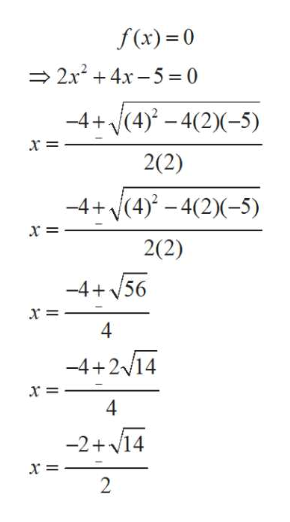# Find the horizontal intercepts as a list of ordered pairs.f(x)=2x2+4x−5

Question
2 views

Find the horizontal intercepts as a list of ordered pairs.

f(x)=2x2+4x−5

check_circle

Step 1

From the sketch shown below, note that on the horizaontal intercepts, y=0. Using this concept we solve the question.

Step 2

Since y is to be equal to 0; f(x)=0help_outlineImage Transcriptionclosef(x) 0 2x4x-5 0 (4)-4(2)5) -4+ 2(2) -4+(4) -4(2)-5) x = 2(2) -4+56 x = 4 -4+214 4 -2+14 2 fullscreen
Step 3

Hence the ordered ...

### Want to see the full answer?

See Solution

#### Want to see this answer and more?

Solutions are written by subject experts who are available 24/7. Questions are typically answered within 1 hour.*

See Solution
*Response times may vary by subject and question.
Tagged in
MathCalculus

### Functions